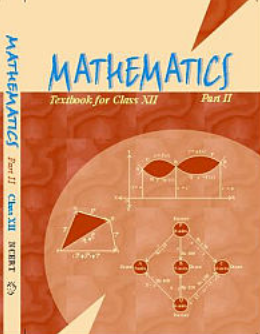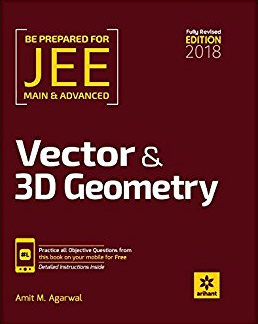# Vector Algebra (Weightage 5%)   Share

### Topics from Vector Algebra

• Vectors and scalars, addition of vectors, components of a vector in two dimensions and three dimensional space (34 concepts)
• Scalar and vector products, scalar and vector triple product (70 concepts)
• Introduction to 3-D Geometry (1 concepts)
• Vector Algebra (9 concepts)
• Scalar and Vector Product of Vector Algebra (11 concepts)

### Important Books for Vector Algebra

••Exams
Articles
Questions